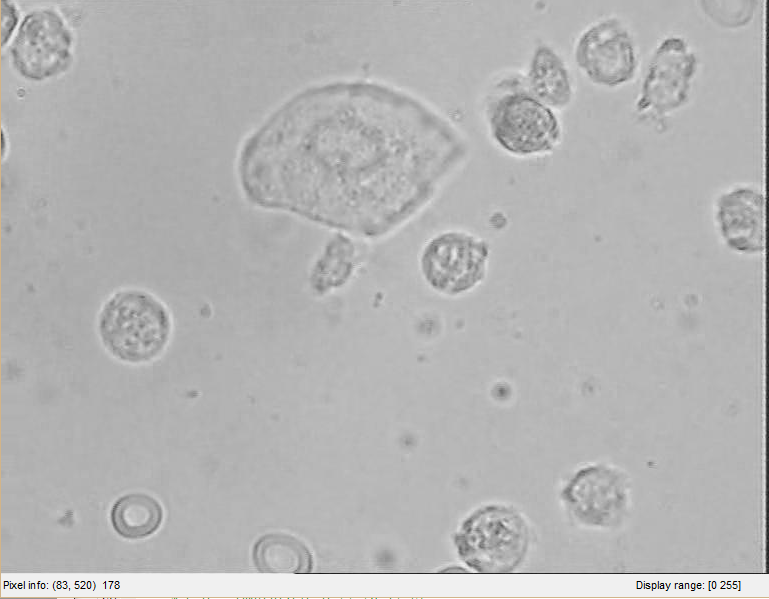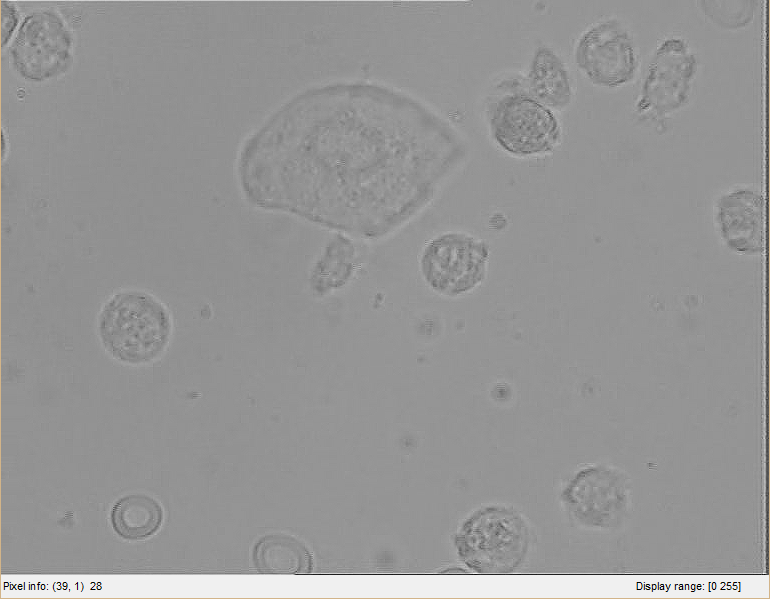## 灰度级的扩展与压缩

```I = imread('2.jpg');
I_g = rgb2gray(I);
imtool(I_g)
% 使用laplacian进行图像锐化，该过程会产生负值，因此需要使用double类型
w = fspecial('laplacian',0);
I_g_d = double(I_g);
g = imfilter(I_g_d,w,'replicate');
I_g_g = I_g_d-g;
imtool(I_g_g,[]);``````y = 255*(I_g_g-min(min(I_g_g)))/(max(max(I_g_g))-min(min(I_g_g)));
y=uint8(y);
imtool(y,[])``````y1=mat2gray(I_g_g); % 将数据压缩到0-1，数据类型仍为double
y1 = im2uint8(y1); % 将数据恢复到0-255，数据类型变为uint8
imtool(y1);```

```I = imread('2.jpg');
I_g = rgb2gray(I);
imtool(I_g)
% 使用laplacian进行图像锐化，该过程会产生负值，因此需要使用double类型
w = fspecial('laplacian',0);
I_g_d = im2double(I_g);
g = imfilter(I_g_d,w,'replicate');
I_g_g = I_g_d-g;
imtool(I_g_g,[]);

y1 = im2uint8(y1);
imtool(y1);```